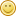# Earth's Curvature on a Smaller Scale

• 4 Replies
• 728 Views
?

#### FlatEarthisStupid

• 29
• I have encountered the sphere-phobes.##### Earth's Curvature on a Smaller Scale
« on: February 20, 2021, 03:00:39 PM »
Some people of the F.E.S ask, "Why can't I look across an Olympic-sized swimming pool and see curvature?". Here's why: there is curvature, but very small, small enough to not be seen with human eyes.

Earth curves at around 8 inches per mile, which means that for every mile, the Earth curves around 8 inches. Dividing 8 by 63,360 (number of inches in a mile), we get 0.000126 inches per inch (rounded). 0.000126 inches is 3.2 micrometers, or 3.2 millionths of a meter. So for every inch, Earth curves 0.0000032 meters or 0.000126 inches. This means for every foot, the Earth curves by 0.001512 inches (0.000126 multiplied by 12), or 38.4 micrometers (0.0000384 meters). An Olympic-sized swimming pool (my example for this) is 164 feet in length (long-course). Multiply 0.001512 by 164 and you get 0.247968 inches, or 6,297.6 micrometers (6.2976 millimeters, or 0.0062976 meters). That's very small. For comparison, a US dime is 0.705 inches in diameter (17.91 millimeters, 0.01791 meters). So no, you wouldn't really be able to see a curvature in an Olympic-sized swimming pool.

Too long, didn't read: An Olympic-sized swimming pool will curve at less than half of the diameter of a US dime, not really visible.
« Last Edit: February 20, 2021, 08:10:07 PM by FlatEarthisStupid »#### Bullwinkle

• The Elder Ones
• 20771
• Standard Idiot##### Re: Earth's Curvature on a Smaller Scale
« Reply #1 on: February 21, 2021, 03:34:25 AM »
would this work on a Walmart parking lot?

?

#### FlatEarthisStupid

• 29
• I have encountered the sphere-phobes.##### Re: Earth's Curvature on a Smaller Scale
« Reply #2 on: February 21, 2021, 06:54:37 AM »
would this work on a Walmart parking lot?

I assume so.##### Re: Earth's Curvature on a Smaller Scale
« Reply #3 on: February 22, 2021, 12:46:52 AM »
Some people of the F.E.S ask, "Why can't I look across an Olympic-sized swimming pool and see curvature?".

Could you please cite a reference? I have not seen that argument before.

Here's why: there is curvature, but very small, small enough to not be seen with human eyes.

Earth curves at around 8 inches per mile, which means that for every mile, the Earth curves around 8 inches.

Wrong. A sphere with a radius of 4000 miles will have a curvature of 8 inches per mile *squared*, not 8 inches per mile.

A surface that "curves" at 8 inches per mile is a slightly tilted flat plane. Congratulations. You've just stated that the Earth is flat.

Dividing 8 by 63,360 (number of inches in a mile), we get 0.000126 inches per inch (rounded).

The division is correct, but the interpretation is correct only if the surface in question is flat.

0.000126 inches is 3.2 micrometers, or 3.2 millionths of a meter. So for every inch, Earth curves 0.0000032 meters or 0.000126 inches.

Again, correct arithmetic, but faulty interpretation.

This means for every foot, the Earth curves by 0.001512 inches (0.000126 multiplied by 12), or 38.4 micrometers (0.0000384 meters).

Nope. The appropriate formula to use for drop of a spherical surface from a point at a distance is (using the approximation when distance << radius)
drop = (2 * distance)^2 / (8 * radius)

For a distance of 1 mile, the drop is 8 inches
For a distance of 1 foot, the drop is 3E-07 inches
For a distance of 1 inch, the drop is 2E-09 inches

An Olympic-sized swimming pool (my example for this) is 164 feet in length (long-course). Multiply 0.001512 by 164 and you get 0.247968 inches, or 6,297.6 micrometers (6.2976 millimeters, or 0.0062976 meters). That's very small.

Your idea of small is relative. With good surveying equipment, at that distance you should be able to see a few thousandths of an inch.

For a distance of 164 feet, the drop is .0077 inches.

For comparison, a US dime is 0.705 inches in diameter (17.91 millimeters, 0.01791 meters). So no, you wouldn't really be able to see a curvature in an Olympic-sized swimming pool.

If that curvature drop was 1/4 inch, I assure you, it would be easily detectable by equipment I have at home.

Too long, didn't read: An Olympic-sized swimming pool will curve at less than half of the diameter of a US dime, not really visible.

So, a full paragraph of nonsensical calculations that have nothing to do with a curved surface, culminating in a conclusion that is totally faulty (that you can't measure 1/4 inch at a distance of 164 feet).

Please tell us again why we should take anything you say seriously?

?

#### JackBlack

• 16862##### Re: Earth's Curvature on a Smaller Scale
« Reply #4 on: February 22, 2021, 01:08:23 AM »
Wrong. A sphere with a radius of 4000 miles will have a curvature of 8 inches per mile *squared*, not 8 inches per mile.

A surface that "curves" at 8 inches per mile is a slightly tilted flat plane. Congratulations. You've just stated that the Earth is flat.
I would say you are both wrong.
But he is more wrong.
Curvature should technically be measured in units of per m or equivalent, based upon natural units of angles (i.e. radians).
The curvature of Earth is thus ~1.6e-7 per m.
This means if you follow it ~40 000 km, you will have curved by 2 pi.

The 8 inches per mile squared is the drop, not the curvature.

And going by who posted it I should have looked at it in more detail. Thanks for pointing out the error.